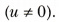# With the help of a graph, derive the relation v = u + at

With the help of a graph, derive the relation v = u + at.

Consider the velocity-time graph of an object that moves under uniform acceleration as shown in the figure